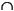MATLAB Function Referenceintersect

Find set intersection of two vectors

Syntax

• ```c = intersect(A, B)
c = intersect(A, B, 'rows')
[c, ia, ib] = intersect(...)
```

Description

```c = intersect(A, B) ``` returns the values common to both A and B. The resulting vector is sorted in ascending order. In set theoretic terms, this is
`A`B. A and B can be cell arrays of strings.

```c = intersect(A, B, 'rows') ``` when `A` and `B` are matrices with the same number of columns returns the rows common to both `A` and `B`.

```[c, ia, ib] = intersect(a, b) ``` also returns column index vectors `ia` and `ib` such that `c = a(ia)` and `c = b(ib)` (or `c = a(ia,:)` and` c = b(ib,:)`).

Examples

• ```A = [1 2 3 6]; B = [1 2 3 4 6 10 20];
[c, ia, ib] = intersect(A, B);
disp([c; ia; ib])
1     2     3     6
1     2     3     4
1     2     3     5
```

See Also

`ismember`, `issorted`, `setdiff`, `setxor`, `union`, `unique`interpstreamspeed intmax© 1994-2005 The MathWorks, Inc.